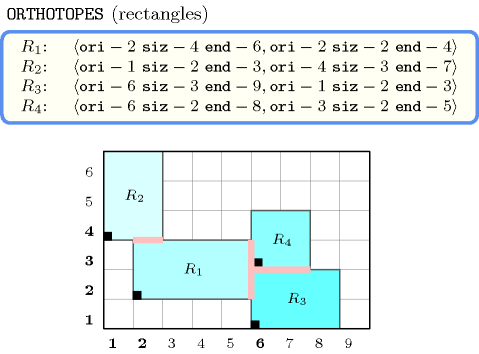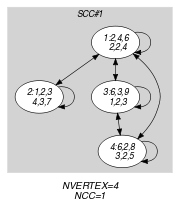## 5.313. orths_are_connected

Origin

N. Beldiceanu

Constraint

$\mathrm{𝚘𝚛𝚝𝚑𝚜}_\mathrm{𝚊𝚛𝚎}_\mathrm{𝚌𝚘𝚗𝚗𝚎𝚌𝚝𝚎𝚍}\left(\mathrm{𝙾𝚁𝚃𝙷𝙾𝚃𝙾𝙿𝙴𝚂}\right)$

Type
 $\mathrm{𝙾𝚁𝚃𝙷𝙾𝚃𝙾𝙿𝙴}$ $\mathrm{𝚌𝚘𝚕𝚕𝚎𝚌𝚝𝚒𝚘𝚗}\left(\mathrm{𝚘𝚛𝚒}-\mathrm{𝚍𝚟𝚊𝚛},\mathrm{𝚜𝚒𝚣}-\mathrm{𝚍𝚟𝚊𝚛},\mathrm{𝚎𝚗𝚍}-\mathrm{𝚍𝚟𝚊𝚛}\right)$
Argument
 $\mathrm{𝙾𝚁𝚃𝙷𝙾𝚃𝙾𝙿𝙴𝚂}$ $\mathrm{𝚌𝚘𝚕𝚕𝚎𝚌𝚝𝚒𝚘𝚗}\left(\mathrm{𝚘𝚛𝚝𝚑}-\mathrm{𝙾𝚁𝚃𝙷𝙾𝚃𝙾𝙿𝙴}\right)$
Restrictions
 $|\mathrm{𝙾𝚁𝚃𝙷𝙾𝚃𝙾𝙿𝙴}|>0$ $\mathrm{𝚛𝚎𝚚𝚞𝚒𝚛𝚎}_\mathrm{𝚊𝚝}_\mathrm{𝚕𝚎𝚊𝚜𝚝}$$\left(2,\mathrm{𝙾𝚁𝚃𝙷𝙾𝚃𝙾𝙿𝙴},\left[\mathrm{𝚘𝚛𝚒},\mathrm{𝚜𝚒𝚣},\mathrm{𝚎𝚗𝚍}\right]\right)$ $\mathrm{𝙾𝚁𝚃𝙷𝙾𝚃𝙾𝙿𝙴}.\mathrm{𝚜𝚒𝚣}>0$ $\mathrm{𝙾𝚁𝚃𝙷𝙾𝚃𝙾𝙿𝙴}.\mathrm{𝚘𝚛𝚒}\le \mathrm{𝙾𝚁𝚃𝙷𝙾𝚃𝙾𝙿𝙴}.\mathrm{𝚎𝚗𝚍}$ $\mathrm{𝚛𝚎𝚚𝚞𝚒𝚛𝚎𝚍}$$\left(\mathrm{𝙾𝚁𝚃𝙷𝙾𝚃𝙾𝙿𝙴𝚂},\mathrm{𝚘𝚛𝚝𝚑}\right)$ $\mathrm{𝚜𝚊𝚖𝚎}_\mathrm{𝚜𝚒𝚣𝚎}$$\left(\mathrm{𝙾𝚁𝚃𝙷𝙾𝚃𝙾𝙿𝙴𝚂},\mathrm{𝚘𝚛𝚝𝚑}\right)$
Purpose

There should be a single group of connected orthotopes. Two orthotopes touch each other (i.e., are connected) if they overlap in all dimensions except one, and if, for the dimension where they do not overlap, the distance between the two orthotopes is equal to 0.

Example
$\left(\begin{array}{c}〈\begin{array}{c}\mathrm{𝚘𝚛𝚝𝚑}-〈\mathrm{𝚘𝚛𝚒}-2\mathrm{𝚜𝚒𝚣}-4\mathrm{𝚎𝚗𝚍}-6,\mathrm{𝚘𝚛𝚒}-2\mathrm{𝚜𝚒𝚣}-2\mathrm{𝚎𝚗𝚍}-4〉,\hfill \\ \mathrm{𝚘𝚛𝚝𝚑}-〈\mathrm{𝚘𝚛𝚒}-1\mathrm{𝚜𝚒𝚣}-2\mathrm{𝚎𝚗𝚍}-3,\mathrm{𝚘𝚛𝚒}-4\mathrm{𝚜𝚒𝚣}-3\mathrm{𝚎𝚗𝚍}-7〉,\hfill \\ \mathrm{𝚘𝚛𝚝𝚑}-〈\mathrm{𝚘𝚛𝚒}-6\mathrm{𝚜𝚒𝚣}-3\mathrm{𝚎𝚗𝚍}-9,\mathrm{𝚘𝚛𝚒}-1\mathrm{𝚜𝚒𝚣}-2\mathrm{𝚎𝚗𝚍}-3〉,\hfill \\ \mathrm{𝚘𝚛𝚝𝚑}-〈\mathrm{𝚘𝚛𝚒}-6\mathrm{𝚜𝚒𝚣}-2\mathrm{𝚎𝚗𝚍}-8,\mathrm{𝚘𝚛𝚒}-3\mathrm{𝚜𝚒𝚣}-2\mathrm{𝚎𝚗𝚍}-5〉\hfill \end{array}〉\hfill \end{array}\right)$

Figure 5.313.1 shows the rectangles associated with the example. One can note that:

• Rectangle 2 touch rectangle 1,

• Rectangle 1 touch rectangle 2, rectangle 3 and rectangle 4,

• Rectangle 4 touch rectangle 1 and rectangle 3,

• Rectangle 3 touch rectangle 1 and rectangle 4.

Consequently, since we have a single group of connected rectangles, the $\mathrm{𝚘𝚛𝚝𝚑𝚜}_\mathrm{𝚊𝚛𝚎}_\mathrm{𝚌𝚘𝚗𝚗𝚎𝚌𝚝𝚎𝚍}$ constraint holds.

##### Figure 5.313.1. The four connected rectangles of the Example slot: contacts between rectangles are shown in pinkTypical
 $|\mathrm{𝙾𝚁𝚃𝙷𝙾𝚃𝙾𝙿𝙴}|>1$ $|\mathrm{𝙾𝚁𝚃𝙷𝙾𝚃𝙾𝙿𝙴𝚂}|>1$
Symmetries
• Items of $\mathrm{𝙾𝚁𝚃𝙷𝙾𝚃𝙾𝙿𝙴𝚂}$ are permutable.

• Items of $\mathrm{𝙾𝚁𝚃𝙷𝙾𝚃𝙾𝙿𝙴𝚂}.\mathrm{𝚘𝚛𝚝𝚑}$ are permutable (same permutation used).

• One and the same constant can be added to the $\mathrm{𝚘𝚛𝚒}$ and $\mathrm{𝚎𝚗𝚍}$ attributes of all items of $\mathrm{𝙾𝚁𝚃𝙷𝙾𝚃𝙾𝙿𝙴𝚂}.\mathrm{𝚘𝚛𝚝𝚑}$.

Usage

In floor planning problem there is a typical constraint, that states that one should be able to access every room from any room.

Keywords
Arc input(s)

$\mathrm{𝙾𝚁𝚃𝙷𝙾𝚃𝙾𝙿𝙴𝚂}$

Arc generator
$\mathrm{𝑆𝐸𝐿𝐹}$$↦\mathrm{𝚌𝚘𝚕𝚕𝚎𝚌𝚝𝚒𝚘𝚗}\left(\mathrm{𝚘𝚛𝚝𝚑𝚘𝚝𝚘𝚙𝚎𝚜}\right)$

Arc arity
Arc constraint(s)
$\mathrm{𝚘𝚛𝚝𝚑}_\mathrm{𝚕𝚒𝚗𝚔}_\mathrm{𝚘𝚛𝚒}_\mathrm{𝚜𝚒𝚣}_\mathrm{𝚎𝚗𝚍}$$\left(\mathrm{𝚘𝚛𝚝𝚑𝚘𝚝𝚘𝚙𝚎𝚜}.\mathrm{𝚘𝚛𝚝𝚑}\right)$
Graph property(ies)
$\mathrm{𝐍𝐀𝐑𝐂}$$=|\mathrm{𝙾𝚁𝚃𝙷𝙾𝚃𝙾𝙿𝙴𝚂}|$

Arc input(s)

$\mathrm{𝙾𝚁𝚃𝙷𝙾𝚃𝙾𝙿𝙴𝚂}$

Arc generator
$\mathrm{𝐶𝐿𝐼𝑄𝑈𝐸}$$\left(\ne \right)↦\mathrm{𝚌𝚘𝚕𝚕𝚎𝚌𝚝𝚒𝚘𝚗}\left(\mathrm{𝚘𝚛𝚝𝚑𝚘𝚝𝚘𝚙𝚎𝚜}\mathtt{1},\mathrm{𝚘𝚛𝚝𝚑𝚘𝚝𝚘𝚙𝚎𝚜}\mathtt{2}\right)$

Arc arity
Arc constraint(s)
$\mathrm{𝚝𝚠𝚘}_\mathrm{𝚘𝚛𝚝𝚑}_\mathrm{𝚊𝚛𝚎}_\mathrm{𝚒𝚗}_\mathrm{𝚌𝚘𝚗𝚝𝚊𝚌𝚝}$$\left(\mathrm{𝚘𝚛𝚝𝚑𝚘𝚝𝚘𝚙𝚎𝚜}\mathtt{1}.\mathrm{𝚘𝚛𝚝𝚑},\mathrm{𝚘𝚛𝚝𝚑𝚘𝚝𝚘𝚙𝚎𝚜}\mathtt{2}.\mathrm{𝚘𝚛𝚝𝚑}\right)$
Graph property(ies)
 $•$$\mathrm{𝐍𝐕𝐄𝐑𝐓𝐄𝐗}$$=|\mathrm{𝙾𝚁𝚃𝙷𝙾𝚃𝙾𝙿𝙴𝚂}|$ $•$$\mathrm{𝐍𝐂𝐂}$$=1$

Graph model

Parts (A) and (B) of Figure 5.313.2 respectively show the initial and final graph associated with the Example slot.Since we use the $\mathrm{𝐍𝐕𝐄𝐑𝐓𝐄𝐗}$ graph property the vertices of the final graph are stressed in bold. Since we also use the $\mathrm{𝐍𝐂𝐂}$ graph property we show the unique connected component of the final graph. An arc between two vertices indicates that two rectangles are in contact.

##### Figure 5.313.2. Initial and final graph of the $\mathrm{𝚘𝚛𝚝𝚑𝚜}_\mathrm{𝚊𝚛𝚎}_\mathrm{𝚌𝚘𝚗𝚗𝚎𝚌𝚝𝚎𝚍}$ constraint(a) (b)
Signature

Since the first graph constraint uses the $\mathrm{𝑆𝐸𝐿𝐹}$ arc generator on the $\mathrm{𝙾𝚁𝚃𝙷𝙾𝚃𝙾𝙿𝙴𝚂}$ collection the corresponding initial graph contains $|\mathrm{𝙾𝚁𝚃𝙷𝙾𝚃𝙾𝙿𝙴𝚂}|$ arcs. Therefore the final graph of the first graph constraint contains at most $|\mathrm{𝙾𝚁𝚃𝙷𝙾𝚃𝙾𝙿𝙴𝚂}|$ arcs and we can rewrite $\mathrm{𝐍𝐀𝐑𝐂}=|\mathrm{𝙾𝚁𝚃𝙷𝙾𝚃𝙾𝙿𝙴𝚂}|$ to $\mathrm{𝐍𝐀𝐑𝐂}\ge |\mathrm{𝙾𝚁𝚃𝙷𝙾𝚃𝙾𝙿𝙴𝚂}|$. So we can simplify $\underline{\overline{\mathrm{𝐍𝐀𝐑𝐂}}}$ to $\overline{\mathrm{𝐍𝐀𝐑𝐂}}$.

Consider now the second graph constraint. Since its corresponding initial graph contains $|\mathrm{𝙾𝚁𝚃𝙷𝙾𝚃𝙾𝙿𝙴𝚂}|$ vertices, its final graph has a maximum number of vertices also equal to $|\mathrm{𝙾𝚁𝚃𝙷𝙾𝚃𝙾𝙿𝙴𝚂}|$. Therefore we can rewrite $\mathrm{𝐍𝐕𝐄𝐑𝐓𝐄𝐗}=|\mathrm{𝙾𝚁𝚃𝙷𝙾𝚃𝙾𝙿𝙴𝚂}|$ to $\mathrm{𝐍𝐕𝐄𝐑𝐓𝐄𝐗}\ge |\mathrm{𝙾𝚁𝚃𝙷𝙾𝚃𝙾𝙿𝙴𝚂}|$ and simplify $\underline{\overline{\mathrm{𝐍𝐕𝐄𝐑𝐓𝐄𝐗}}}$ to $\overline{\mathrm{𝐍𝐕𝐄𝐑𝐓𝐄𝐗}}$. From the graph property $\mathrm{𝐍𝐕𝐄𝐑𝐓𝐄𝐗}=|\mathrm{𝙾𝚁𝚃𝙷𝙾𝚃𝙾𝙿𝙴𝚂}|$ and from the restriction $|\mathrm{𝙾𝚁𝚃𝙷𝙾𝚃𝙾𝙿𝙴𝚂}|>0$ the final graph is not empty. Therefore it contains at least one connected component. So we can rewrite $\mathrm{𝐍𝐂𝐂}=1$ to $\mathrm{𝐍𝐂𝐂}\le 1$ and simplify $\underline{\overline{\mathrm{𝐍𝐂𝐂}}}$ to $\underline{\mathrm{𝐍𝐂𝐂}}$.